# Logic

Now let’s talk about one more seemingly unrelated topic just so we can “surprise” ourselves when we realize it’s category theory. By the way, in this chapter there will be another surprise in addition to that, so don’t fall asleep.

Also, I will not merely transport you to a different branch of mathematics, but to an entirely different discipline - logic.

# What is logic

Logic is the science of the possible. As such, it is at the root of all other sciences, all of which are sciences of the actual, i.e. that which really exists. For example, if science explains how our universe works then logic is the part of the description which is also applicable to any other universe that is possible to exist. A scientific theory aims to be consistent with both itself and observations, while a logical theory only needs to be consistent with itself.

Logic studies the rules by which knowing one thing leads you to conclude (or prove) that some other thing is also true, regardless of the things’ domain (e.g. scientific discipline) and by only referring to their form.

On top of that, it (logic) tries to organize those rules in logical systems (or formal systems as they are also called).

## Logic and mathematics

Seeing this description, we might think that the subject of logic is quite similar to the subject of set theory and category theory, as we described it in the first chapter - instead of the word “formal” we used another similar word, namely “abstract”, and instead of “logical system” we said “theory”. This observation would be quite correct - today most people agree that every mathematical theory is actually logic plus some additional definitions added to it. For example, part of the reason why set theory is so popular as a theory for the foundations of mathematics is that it adds just one single primitive to the standard axioms of logic which we will see shortly - the binary relation that indicates set membership. Category theory is close to logic too, but in a quite different way.

## Primary propositions

A consequence of logic being the science of the possible is that in order to do anything at all in it, we should have an initial set of propositions that we accept as true or false. These are also called “premises”, “primary propositions” or “atomic propositions” as Wittgenstein dubbed them.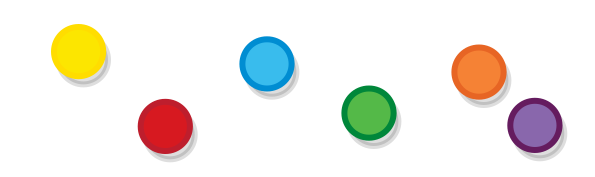In the context of logic itself, these propositions are abstracted away (i.e. we are not concerned about them directly) and so they can be represented with the colorful balls that you are familiar with.

## Composing propositions

At the heart of logic, as in category theory, is the concept of composition - if we have two or more propositions that are somehow related to one another, we can combine them into one using a logical operators, like “and”, “or” “follows” etc. The result would be a new proposition, not unlike the way in which two monoid objects are combined into one using the monoid operation. And actually some logical operations do form monoids, like for example the operation and, with the proposition true serving as the identity element.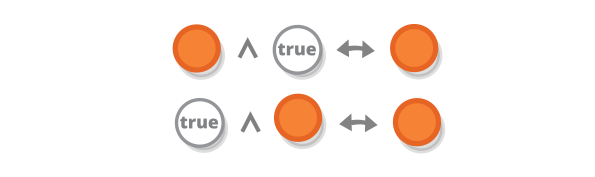However, unlike monoids/groups, logics have not one but many logical operations and logic studies the ways in which they relate to one another, for example, in logic we might be interested in the law of distributivity of and and or operations and what it entails.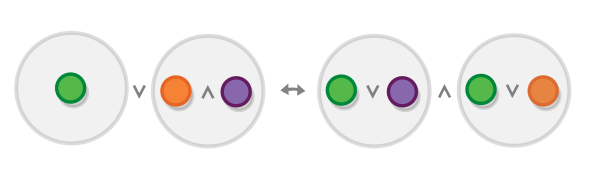Important to note that is the symbol for and and is the symbol for or (although the law above is actually valid even if and and or are flipped).

## The equivalence of primary and composite propositions

When looking at the last diagram, it is important to stress that, although in the leftmost proposition the green ball is wrapped in a gray ball to make the diagram prettier, propositions that are composed of several premises (symbolized by gray balls, containing some other balls) are not in any way different from “primary” propositions (single-color balls) and that they compose in the same way.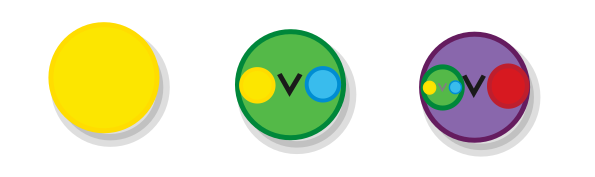## Modus ponens

As an example of a proposition that contains multiple levels of nesting (and also as a great introduction of the subject of logic in its own right), consider one of the oldest (it was already known by Stoics at 3rd century B.C.) and most famous propositions ever, namely the modus ponens.

Modus ponens is a proposition that states that if proposition A is true and also if proposition (A → B) is true (that is if A implies B), then B is true as well. For example, if we know that “Socrates is a human” and that “humans are mortal” (or “being human implies being mortal”), we also know that “Socrates is mortal.”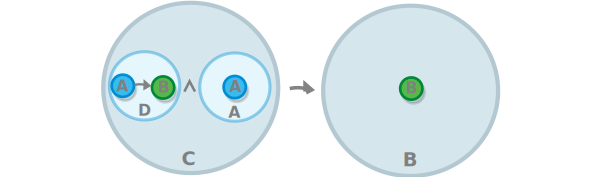Let’s dive into it. The proposition is composed of two other propositions in a follows relation, where the proposition that follows (B) is primary, but the proposition from which B follows is not primary (let’s call that one C - so the whole proposition becomes C → B.)

Going one more level down, we notice that the C propositions is itself composed of two propositions in an and, relationship - A and let’s call the other one D (so A ∧ D), where D is itself composed of two propositions, this time in a follows relationship - A → B. But all of this is better visualized in the diagram.

## Tautologies

We often cannot tell whether a given composite proposition is true or false without knowing the values of the propositions that compose it. However, with propositions such as modus ponens we can: modus ponens is always true, regardless of whether the propositions that form it are true or false. If we want to be fancy, we can also say that it is true in all models of the logical system, a model being a set of real-world premises are taken to be signified by our propositions.

For example, our previous example will not stop being true if we substitute “Socrates” with any other name, nor if we substitute “mortal” for any other quality that humans possess.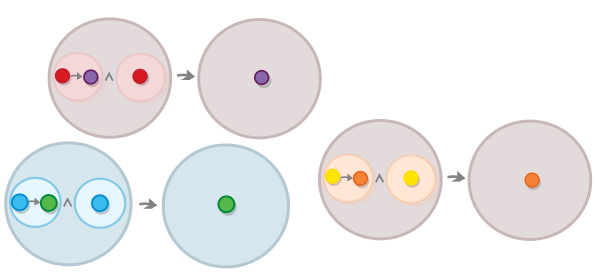Propositions that are always true are called tautologies. And their more-famous counterparts that are always false are called contradictions. You can turn each tautology into contradiction or the other way around by adding a “not”.

The simplest tautology is the statement that each proposition implies itself (e.g. “All bachelors are unmarried”). It may remind you of something.Here are some more complex (less boring) tautologies (the symbol ¬ means “not”/negation.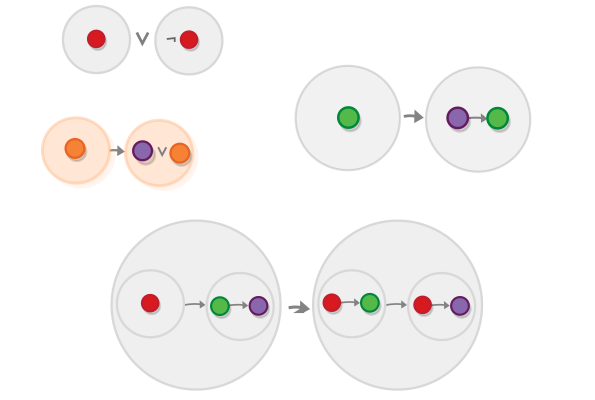We will learn how to determine which propositions are a tautologies shortly, but first let’s see why is this important at all i.e. what are tautologies good for: tautologies are useful because they are the basis of axiom schemas/rules of inference. And axiom schemas or rules of inference serve as starting point from which we can generate other true logical statements by means of substitution.

## Axiom schemas/Rules of inference

Realizing that the colors of the balls in modus ponens are superficial, we may want to represent the general structure of modus ponens that all of its variations share.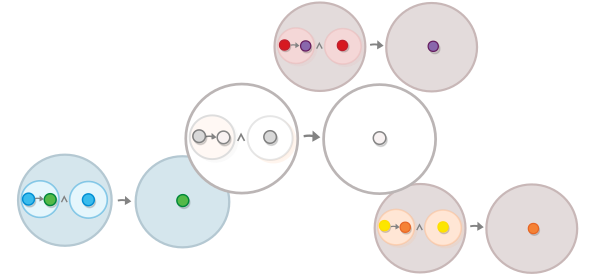This structure (the one that looks like a coloring book in our example) is called axiom schema. And the propositions that are produced by it are axioms.

Note that the propositions that we plug into the schema don’t have to be primary. For example, having the proposition a (that is symbolized below by the orange ball) and the proposition stating that a implies a or b (which is one of the tautologies that we saw above), we can plug those propositions into the modus ponens and prove that a or b is true.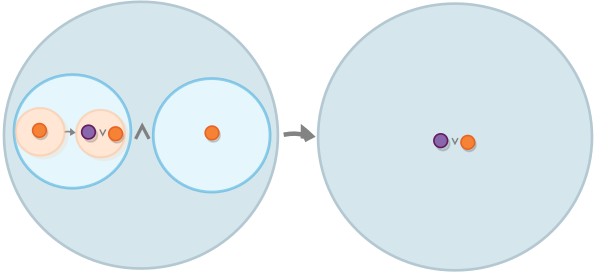This is it about axiom schemas and *rules of inference are almost the same thing except they allow us to actually distill the conclusion from the premises. For example in the case above, we can use modus ponens as a rule of inference to proves that a or b is true.

All axiom schemas can be easily applied as rules of inference and the other way around.

## Logical systems

Knowing that we can use axiom schemas/rules of inference to generate new propositions, we might ask whether it is possible to create a small collection of such schemas/rules that is curated in such a way that it enables us to generate all other propositions. You would be happy (although a little annoyed, I imagine) to learn that there exist not only one, but many such collections. And yes, collections of this sort are what we call logical systems.

Here is one such collection which consists of the following five axiom schemes in addition to the inference rule modus ponens (These are axiom schemes, even though we use colors).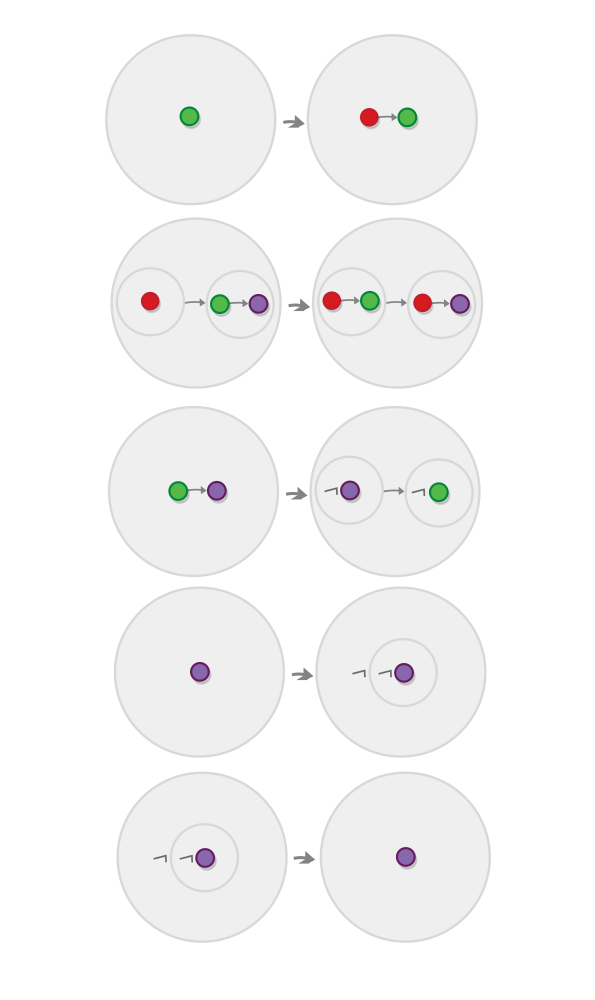Proving that this and other similar logical systems are complete (can really generate all other propositions) is due to Gödel and is known as “Gödel’s completeness theorem” (Gödel is so important that I specifically searched for the ö letter so I can spell his hame right.)

## Conclusion

We now have an idea about how do some of the main logical constructs (axioms, rules of inference) work. But in order to prove that they are true, and to understand what they are, we need to do so through a specific interpretation of those constructs.

We will look into two interpretations - one very old and the other, relatively recent. This would be a slight detour from our usual subject matter of points and arrows, but I assure you that it would be worth it. So let’s start.

# Classical logic. The truth-functional interpretation

Beyond the world that we inhabit and perceive every day, there exist the world of forms where reside all ideas and concepts that manifest themselves in the objects that we perceive e.g. beyond all the people that have ever lived, there lies the prototypical person, and we are people only insofar as we resemble that person, beyond all the things in the world that are strong, lies the ultimate concepts of strength, from which all of them borrow etc. And although, as mere mortals, we live in the world of appearances and cannot perceive the world of forms, we can, through philosophy, “recollect” with it and know some of its features.

The above is a summary of a worldview that is due to the Greek philosopher Plato and is sometimes called Plato’s theory of forms. Originally, the discipline of logic represents an effort to think and structure our thoughts in a way that they apply to this world of forms i.e. in a “formal” way. Today, this original paradigm of logic is known as “classical logic”. Although it all started with Plato, most of it is due to the 20th century mathematician David Hilbert.

The existence of the world of forms implies that even if there are many things that we people don’t know, at least somewhere out there there exists an answer to every question. In logic, this translates to the principle of bivalence that states that each proposition is either true or false. Due to this principle, propositions in classical logic can be aptly represented using set theory by boolean set, which contains those two values.According to the classical interpretation, you can think of primary propositions as just a bunch of boolean values, logical operators are functions that take a one or several boolean values and return another boolean value (and composite propositions are, just the results of the application of these functions).

Let’s review all logical operators in this context.

## The negation operation

Let’s begin with the negation operation. Negation is a unary operation, which means that it is a function that takes just one argument and (like all other logical operators) returns one value, where both the arguments and the return type are boolean values.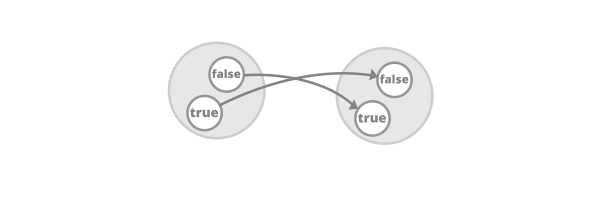The same function can also be expressed in a slightly less-fancy way by this table.

p ¬p
True False
False True

Tables like this one are called truth tables and they are ubiquitous in classical logic. They can be used not only for defining operators but for proving results as well.

## Interlude: Proving results by truth tables

Having defined the negation operator, we are in position to prove the first of the axioms of the logical system we saw, namely the double negation elimination. In natural language, this axiom is equivalent to the observation that saying “I am not unable to do X” is the same as saying “I am able to do it”.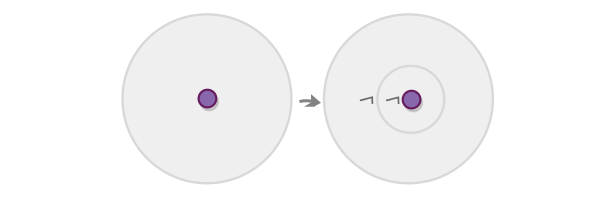(despite its triviality, the double negation axiom is probably the most controversial result in logic, we will see why later.)

If we view logical operators as functions from and to the set of boolean values, than proving axioms involves composing several of those functions into one function and observing its output. More specifically, the proof of the formula above involves just composing the negation function with itself and verifying that it leaves us in the same place from which we started.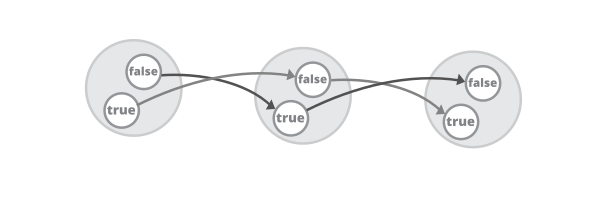If we want to be formal about it, we might say that applying negation two times is equivalent to applying the identity function.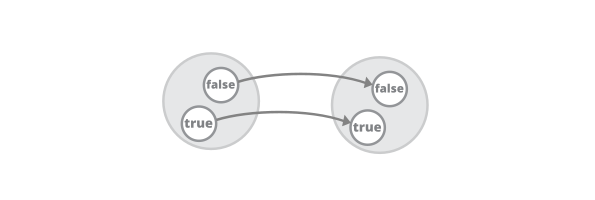If we are tired of diagrams, we can represent the composition diagram above as table as well.

p ¬p ¬¬p
True False True
False True False

Each proposition in classical logic can be proved with such diagrams/tables.

## The and and or operations

OK, you know what and means and I know what it means, but what about those annoying people that want everything to be formally specified (nudge, nudge). Well we already know how we can satisfy them - we just have to construct the boolean function that represents and.

Because and is a binary operator, instead of a single value the function would accept a pair of boolean values.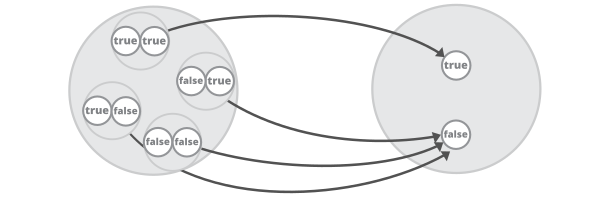Here is the equivalent truth-table (in which is the symbol for and.)

p q p ∧ q
True True True
True False False
False True False
False False False

We can do the same for or, here is the table.

p q p ∨ q
True True True
True False True
False True True
False False False

Task: Draw the diagram for or.

Using those tables, we can also prove some axiom schemas we can use later:

• For and: p ∧ q → p and p ∧ q → q “If I am tired and hungry, this means that I am hungry”.
• For or: p → p ∨ q and p → p ∨ q “If I have a pen this means that I am either have a pen or a ruler”.

## The implies operation

Let’s now look into something less trivial: the implies operation, (also known as entailment). This operation binds two propositions in a way that the truth of the first one implies the truth of the second one. You can read p → q as “if p is true, then q must also be true.

Entailment is also a binary function - it is represented by a function from an ordered pair of boolean values, to a boolean value.

p q p → q
True True True
True False False
False True True
False False True

Now there are some aspects of this which are non-obvious so let’s go through every case.

1. If p is true and q is also true, then p does imply q - obviously.
2. If p is true but q is false then q does not follow from p - cause q would have been true if it did.
3. If p is false but q is true, then p still does imply q. What the hell? Consider that by saying that p implies q we don’t say that the two are 100% interdependent e.g. the claim that “drinking alcohol causes headache” does not mean that drinking is the only source of headaches.
4. And finally if p is false but q is false too, then p still does imply q (just some other day).

It might help you to remember that in classical logic p → q (p implies q) is true when -p ∨ q (either p is false or q is true.)

## Proving results by axioms/rules of inference

Let’s examine the above formula, stating that p → q is the same as ¬p ∨ q.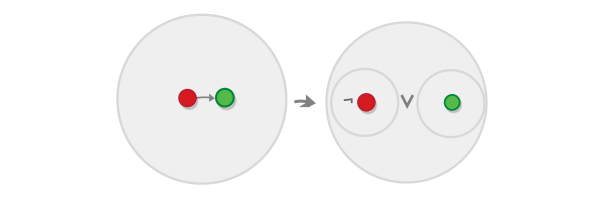We can easily prove this by using truth tables.

p q p → q ¬p q ¬p ∨ q
True True True False True True
True False False False False False
False True True True True True
False False True True False True

But it would be much more intuitive if we do it using axioms and rules of inference. To do so, we have to start with the formula we have (p → q) plus the axiom schemas, and arrive at the formula we want to prove (¬p ∨ q).

Here is one way to do it. The formulas that are used at each step are specified at the right-hand side, the rule of inference is modus ponens.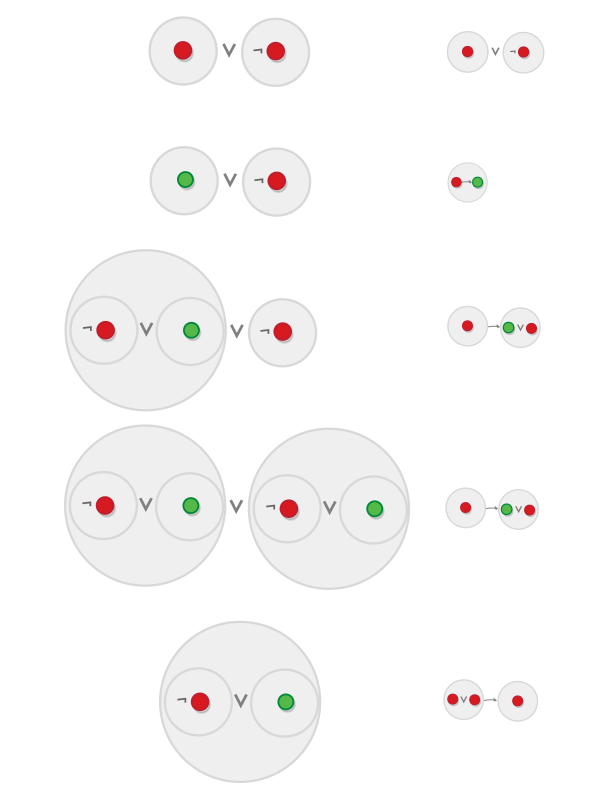Note that to really prove that the two formulas are equivalen we have to also do it the other way around (start with (¬p ∨ q) and (p → q)).

# Intuitionistic logic. The BHK interpretation

Although the classical truth-functional interpretation of logic works and is correct in its own right, it doesn’t fit well the categorical framework that we are using here: It is too “low-level”, it relies on manipulating the values of the propositions. According to it, the operations and, or and implies are just 3 of the 16 possible binary logical operations and they are not really connected to each other.

For these and other reasons (mostly other, probably), in the 20th century a whole new school of logic was founded, called intuitionistic logic. If we view classical logic as based on set theory, then intuitionistic logic would be based on category theory and its related theories. If classical logic is based on Plato’s theory of forms, then intuinism began with a philosophical idea originating from Kant and Schopenhauer: the idea that the world as we experience it is largely predetermined of out perceptions of it. As the mathematician L.E.J. Brouwer puts it.

[…] logic is life in the human brain; it may accompany life outside the brain but it can never guide it by virtue of its own power.

Classical and intuitionistic logic diverge from one another right from the start: because according to intuitionistic logic we are constructing proofs rather than discovering them or unveiling a universal truth, we are off with the principle of bivalence, that is, we have no basis to claim that each statements is necessarily true or false. For example, there might be a statements that might not be provable not because they are false, but simply because they fall outside of the domain of a given logical system (the twin-prime conjecture is often given as an example for this.)

So, intuitionistic logic is not bivalent, i.e. we cannot have all propositions reduced to true and false.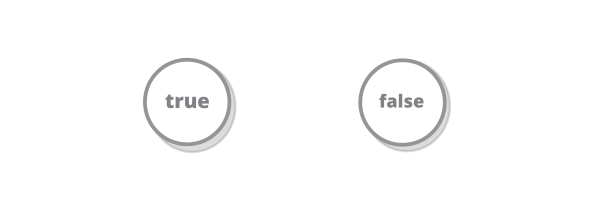One thing that we still do have there are propositions that are “true” in the sense that a proof for them is given - the primary propositions. So with some caveats (which we will see later) the bivalence between true and false proposition might be thought out as similar to the bivalence between the existence or absense of a proof for a given proposition - there either is a proof of it or there isn’t.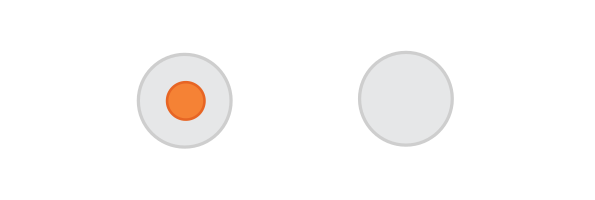This bivalence is at the heart of what is called the Brouwer–Heyting–Kolmogorov (BHK) interpretation of logic, something that we will look into next.

The original formulation of the BHK interpretation is not based on any particular mathematical theory. Here, we will first illustrate it using the language of set theory (just so we can abandon it a little later).

## The and and or operations

As the existence of a proof of a proposition is taken to mean that the proposition is true, the definitions of and is rather simple - the proof of A ∧ B is just a pair containing a proof of A, and a proof of B i.e. a set-theoretic product of the two (see chapter 2). The principle for determining whether the proposition is true or false is similar to that of primary propositions - if the pair of proofs of A and B exist (i.e. if both proofs exist) then the proof of A ∧ B can be constructed (and so A ∧ B is “true”).Question: what would be the or operation in this case?

## The implies operation

Saying that A implies B (A → B) would just mean that there exist a function which can convert a proof of A to a proof of B.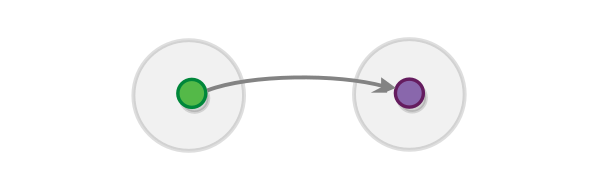And the modus ponens rule of inference is expressed by the fact that if we have a proof of A we can call this function (A → B) to obtain a proof of B.

(Note that in order for this to work, we also need to define the functions in terms of sets i.e. we need to have a set representing A → B for each A and B. We will come back to this later.)

## The negation operation

So according to BHK interpretation saying that A is true, means that that we possess a proof of A - simple enough. But it’s a bit harder to express the fact that A is false: it is not enough to say that we don’t have a proof of A (the fact that don’t have it, doesn’t mean it doesn’t exist). Instead, we must show that claiming that A is true leads to a contradiction.

To express this, intuitionistic logic defines the constant which plays the role of False (and is also known as “absurdity” or “bottom value”). is defined as the proof of a formula that does not have any proofs. And the equivalent of false propositions are the ones that imply that the bottom value is provable (which is a contradiction). So ¬A is A → ⊥.

In set theory, the constant is expressed by the empty set.And the observation that propositions that are connected to the bottom value are false is expressed by the fact that if a proposition is true, i.e. there exists a proof of it, then there can be no function from it to the empty set.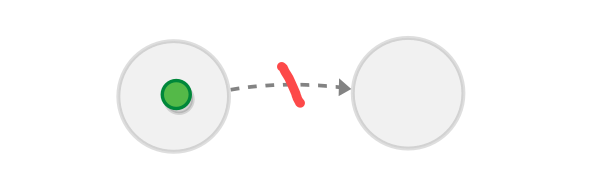The only way for there to be such function is if the set of proofs of the proposition is empty as well.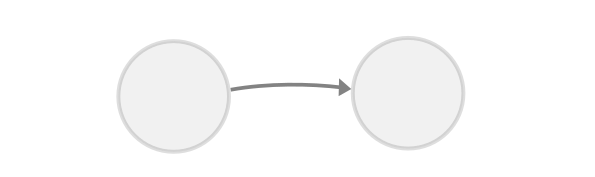Task: Look up the definition of function and verify that there cannot exist a function from any set to the empty set

Task Look up the definition of function and verify that there does exist a function from the empty set to itself (in fact there exist a function from the empty set to any other set.

## Classical VS intuitionistic logic

Although from first glance intuitionistic logic seems to differ a lot from classical logic, it actually doesn’t - if we try to deduce the axiom schemas/rules of inference that correspond to the definitions of the structures outlined above, we would see that they are virtually the same as the ones that define classical logic. With one exception concerning the double negation elimination axiom that we saw earlier, a version of which is known as the law of excluded middle.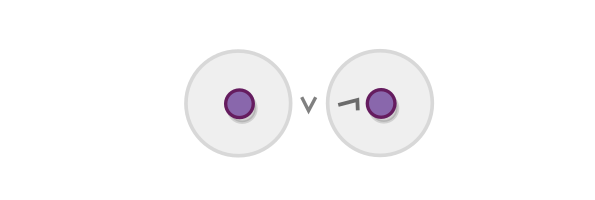This law is valid in classical logic and is true when we look at it in terms of truth tables, but there is no justification for it in the BHK interpretation - a fact that spawned a heated debate between the inventor of classical logic David Hilbert and the inventor of intuitionistic logic L.E.J. Brouwer, known as the Brouwer–Hilbert controversy.

# Logics as categories

Leaving the differences between intuitionistic and classical logics aside, the BHK interpretation is interesting because it provides that higher-level view of logic, that we need in order to construct a interpretation of it based on category theory.

Such higher-level interpretations of logic are sometimes called algebraic interpretations, algebraic being an umbrella term describing all structures that can be represented using category theory, like groups and orders.

## The Curry-Howard isomorphism

Programmers might find the definition of the BHK interpretation interesting for other reason - it is very similar to a definition of a programming language: propositions are types, the implies operations are functions, and operations are composite types (objects), and or operations are sum types (which are currently not supported in most programming languages, but that’s a separate topic.) Finally a proof of a given proposition is represented by a value of the corresponding type.This similarity is known as the Curry-Howard isomorphism.

Task: The Curry-Howard isomorphism is also the basis of special types of programming languages called “proof assistants” which help you verify logical proofs. Install a proof assistant and try to see how it works (I recommend the Coq Tutorial by Mike Nahas).

## Cartesian closed categories

Knowing about the Curry-Howard isomorphism and knowing also that programming languages can be described by category theory may lead us to think that category theory is part of this isomorphism as well. And we would be quite correct, this is why it is sometimes known as the Curry-Howard-Lambek isomorphism, Lambek being the person who discovered the categorical side.

Let’s examine this isomorphism (without being too formal about it). As all other isomorphisms, it comes in two parts. The first part is finding a way to convert a logical system into a category - this would not be hard for us, as sets form a category and the flavor of the BHK interpretation that we saw is based on sets.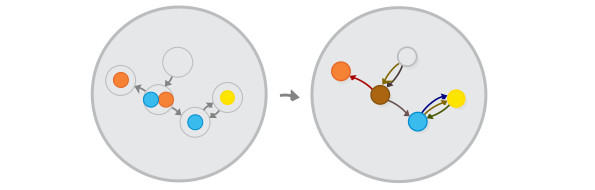Task: See whether you can prove that logic propositions and entailments forms a category. What is missing?

The second part involves converting a category into a logical system - this is much harder. To do it, we have to enumerate the criteria that a given category has to adhere to, in order for it to be “logical”. These criteria have to guarantee that the category has objects that correspond to all valid logical propositions and no objects that correspond to invalid ones.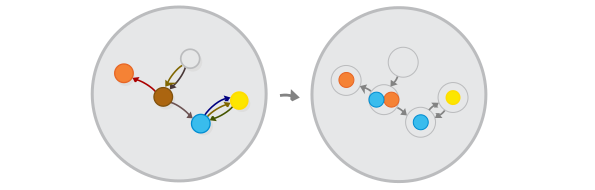Categories that adhere to these criteria are called cartesian closed categories. We won’t describe them here directly, but instead we would start with a similar but simpler structures that are instance of them and that we already examined - orders.

## Logics as orders

We will now do something that is quite characteristic of category theory - examining a concept in a more limited version of the theory, in order to make things simpler for ourselves.

So we already saw that a logical system along with a set of primary propositions forms a category.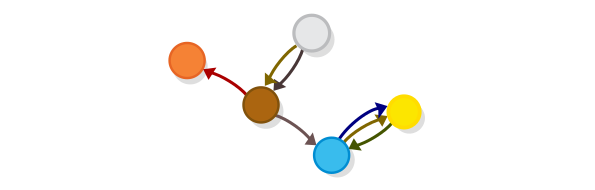If we assume that there is only one way to go from proposition A, to proposition B (or there are many ways, but we are not interested in the difference between them), then logic is not only a category, but a preorder in which the relationship “bigger than” is taken to mean “implies”.Furthermore, if we count propositions that follow from each other (or sets of propositions that are proven by the same proof) as equivalent, then logic is a proper partial order.And so it can be represented by a Hasse diagram, yey.Now let’s examine the question that we asked before - exactly which categories orders represent logic and what laws does an order have to obey so it is isomorphic to a logical system? We will attempt to answer this question as we examine the elements of logic again, this time in the context of orders.

## The and and or operations

By now you probably realized that the and and or operations are the bread and butter of logic (although it’s not clear which is which). As we saw, in the BHK interpretation those are represented by set products and sums. The equivalent constructs in the realm of order theory are meets and joins (in category-theoretic terms products and coproducts.)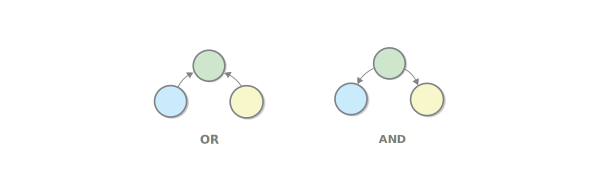Here comes the first criteria for an order to represent a logical system accurately - it has to have meet and join operations for all elements. Having two elements without a meet would mean that you would have a logical system where there are propositions for which you cannot say that one or the other is true. And this not how logic works, so our order has to have meets and joins for all elements. Incidentally we already know how such orders are called - they are called lattices.

One important law of the and and or operations, that is not always present in the meet-s and join-s concerns the connection between the two, i.e. way that they distribute, over one another.Lattices that obey this law are called distributive lattices.

Wait, where have we heard about distributive lattices before? In the previous chapter we said that they are isomorphic to inclusion orders i.e. orders which contain all combinations of sets of a given number of elements. The fact that they popped up again is not coincidental - “logical” orders are isomorphic to inclusion orders. To understand why, you only need to think about the BHK interpretation - the elements which participate in the inclusion are our prime propositions. And the inclusions are all combinations of these elements, in an or relationship (for simplicity’s sake, we are ignoring the and operation.)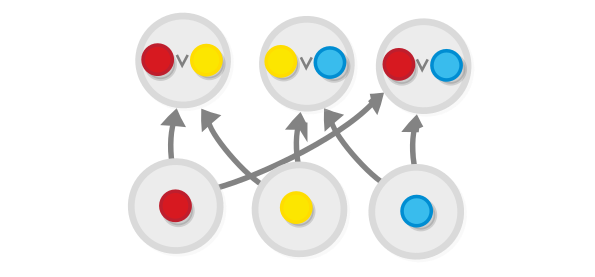NB: For historical reasons, the symbols for and and or logical operations are flipped when compared to arrows in the diagrams ∧ is and and ∨ is or.

## The negation operation

In order for a distributive lattice to represent a logical system, it has to also have objects that correspond to the values True and False. But to mandate that these objects exist, we must first find a way to specify what they are in order/category-theoretic terms.

A well-known result in logic, called the principle of explosion, states that if we have a proof of False (or if “False is true” if we use the terminology of classical logic), then any and every other statement can be proven. And we also know that no true statement implies False (in fact in intuinistic logic this is the definition of a true statement). Based on these criteria we know that the False object would look like this when compared to other objects: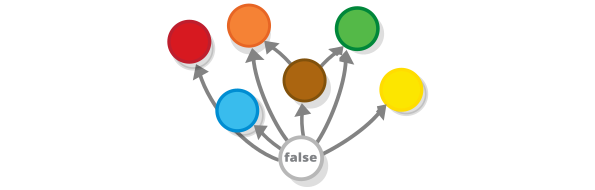Circling back to the BHK interpretation, we see that the empty set fits both conditions.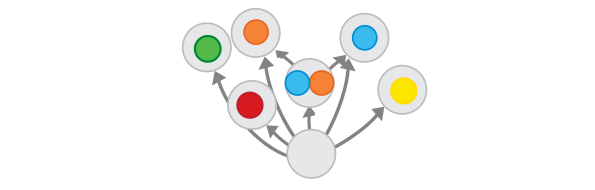Conversely, the proof of True (or the statement that “True is true”) is trivial and doesn’t say anything, so nothing follows from it, but at the same time it follows from every other statement.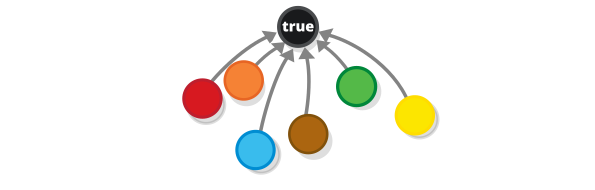So True and False are just the greatest and least objects of our order (in category-theoretic terms terminal and initial object.)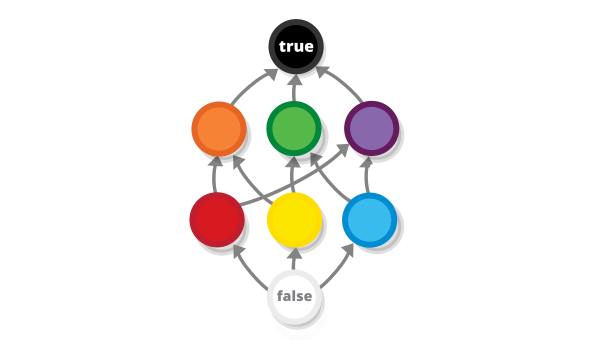This is another example of the categorical concept of duality - True and False are dual to each other, (which makes a lot of sense if you think about it).

So in order to represent logic, our distributive lattice has to also be bounded i.e. it has to have greatest and least elements (which play the roles of True and False).

## The implies operation

Finally, if a lattice really represents a logical system (that is, it is isomorphic to a set of propositions) it also has to have function objects i.e. there needs to be a rule that identifies a unique object A → B for each pair of objects A and B, such that all axioms of logic are followed.

How would this object be described? You guessed it, using categorical language i.e. by recognizing a structure that consists of set of relations between objects in which (A → B) plays a part.This structure is actually a categorical reincarnation our favorite rule of inference, the modus ponens (A ∧ (A → B) → B). This rule is the essence of the implies operation and, because we already know how the operations that it contains (and and implies) are represented in our lattice, we can directly “categorize” it and use it as a definition, saying that (A → B) is the object which is related to objects A and B in such a way that such that A ∧ (A → B) → B.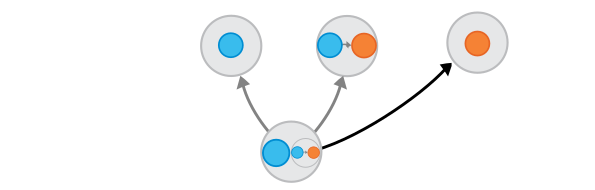This definition is not complete, however, because (A → B) is not the only object that fits in this formula. For example, the set A → B ∧ C is also one such object, as is A → B ∧ C ∧ D. So how do we set apart the real formula from all those “imposter” formulas? If you remember the definitions of the categorical product (or of it’s equivalent for orders, the meet operation) you would already know where this is going: we define the function object using a universal property, by recognizing that all other formulas that can be in the place of X in A ∧ X → B point to (A → B) i.e. they are below (A → B) in a Hasse diagram.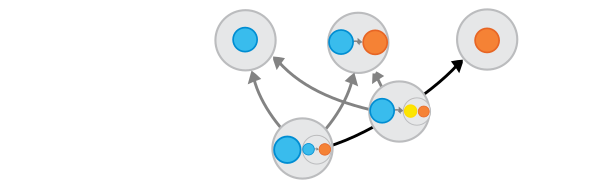Or, using the logic terminology, we say that A → B ∧ C and A → B ∧ C ∧ D etc. are all “stronger” results than (A → B) and so (A → B) is the weakest result that fits the formula (stronger results lay lower in the diagram).

So this is the final condition for an order/lattice to be a representation of logic - for each pair A and B, it has to have a unique object X which obey the formula A ∧ X → B and the universal property. In category theory this object is called the exponential object.

Without being too formal, let’s try to test if this definition captures the concept correctly by examining a few special cases.

For example, let’s take A and B to be the same object. In this case, (A → B) (or (A → A) if you want to be pedantic) would be the topmost object X for which the criteria given by the formula A ∧ X → A is satisfied. But in this case the formula is always satisfied as the meet of A and any other object would always be below A. So this formula is always for all X. The topmost object that fits it is, then, the topmost object out there i.e. True.This corresponds to the identity axiom in logic, that states that everything follows from itself.

And by the similar logic we can see easily that if we take A to be any object that is below B, then (A → B) will also correspond to the True object.So if we have A → B if A implies B, then (A → B) is always true.

And what if B is lower than A. In this case the topmost object that fits the formula A ∧ X → B is B itself: B fits the formula because the meet of two objects is always below those same objects, so A ∧ B → B for all A and B. And B is definitely the topmost object that can possibly fit it, as it literary sets its upper bound.Translated to logical language, says that if B → A, then the proof of (A → B) is just the proof of B.

Note that this definition does not follow the one from the truth tables exactly. This is because this definition is valid specifically for intuinistic logic. For classical logic, the definition of (A → B) is simpler - it is just equivalent to (-A ∨ B).

By the way, the law of distributivity follows from this criteria, so the only criteria that are left for an lattice to follow the laws of intuinistic logic is for it to be bounded i.e. to have greatest and least objects (True and False) and to have a function object as described above. Lattices that follow these criteria are called Heyting algrebras.

And for a lattice to follow the laws of classical logic it has to be bounded and distributive and to be complemented which is to say that each proposition A should be complemented with a unique proposition -A (such that A ∨ -A = 1 and A ∧ -A = 0). These lattices are called Boolean algebras.